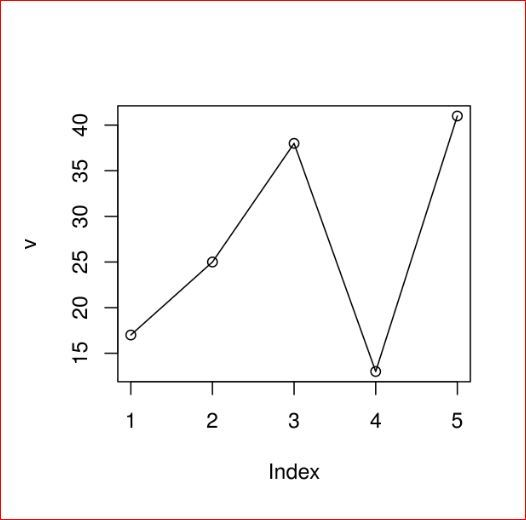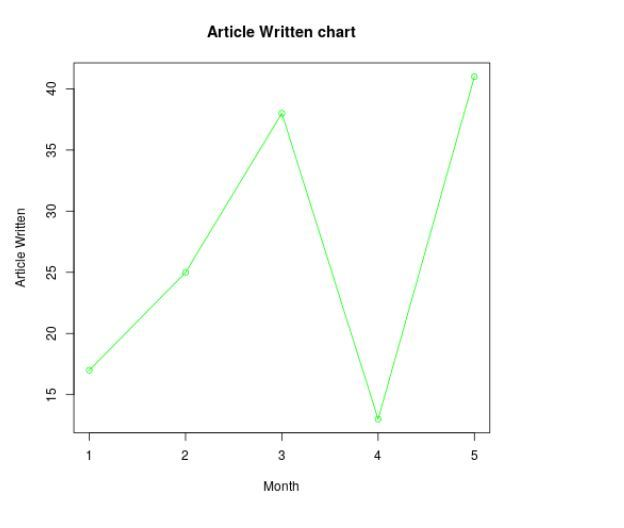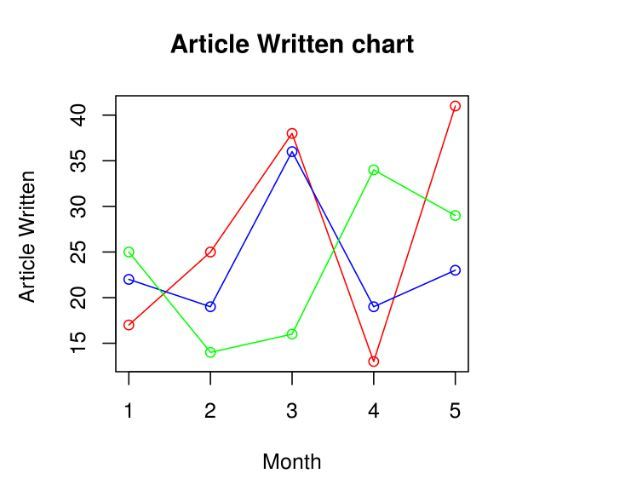GFG App
Open AppBrowser
Continue

A line graph is a chart that is used to display information in the form of a series of data points. It utilizes points and lines to represent change over time. Line graphs are drawn by plotting different points on their X coordinates and Y coordinates, then by joining them together through a line from beginning to end. The graph represents different values as it can move up and down based on the suitable variable.

## R – Line Graphs

The plot() function in R is used to create the line graph.

Syntax: plot(v, type, col, xlab, ylab)

Parameters:

• v: This parameter is a contains only the numeric values
• type: This parameter has the following value:
1. “p” : This value is used to draw only the points.
2. “l” : This value is used to draw only the lines.
3. “o”: This value is used to draw both points and lines
• xlab: This parameter is the label for x axis in the chart.
• ylab: This parameter is the label for y axis in the chart.
• main: This parameter main is the title of the chart.
• col: This parameter is used to give colors to both the points and lines.

### Creating a Simple Line Graph

Approach: In order to create a line graph:

1. It is created using the type parameter as “o” and input vector.
2. Below code to describe the line graph.

Example:

## R

 `# Create the data for the chart.` `v <- ``c``(17, 25, 38, 13, 41)`   `# Plot the bar chart.` `plot``(v, type = ``"o"``)`

Output:## Adding Title, Color and Labels in Line Graphs in R

Approach: To create a colored and labeled line chart.

1. Take all parameters which are required to make line chart by giving a title to the chart and add labels to the axes.
2. We can add more features by adding more parameters with more colors to the points and lines.

Example:

## R

 `# Create the data for the chart.` `v <- ``c``(17, 25, 38, 13, 41)`   `# Plot the bar chart.` `plot``(v, type = ``"o"``, col = ``"green"``,` `    ``xlab = ``"Month"``, ylab = ``"Article Written"``,` `    ``main = ``"Article Written chart"``)`

Output:## Multiple Lines in a Line Graph in R Programming Language

Approach: To create multiple line graphs.

1. In above example, we created line graphs by only one line in each graph.
2. Now creating multiple lines to describe it more clearly.

Example:

## R

 `# Create the data for the chart.` `v <- ``c``(17, 25, 38, 13, 41)` `t <- ``c``(22, 19, 36, 19, 23)` `m <- ``c``(25, 14, 16, 34, 29)`   `# Plot the bar chart.` `plot``(v, type = ``"o"``, col = ``"red"``,` `    ``xlab = ``"Month"``, ylab = ``"Article Written "``,` `    ``main = ``"Article Written chart"``)`   `lines``(t, type = ``"o"``, col = ``"blue"``)` `lines``(m, type = ``"o"``, col = ``"green"``)`

Output: When we execute the above code, it shows the following result-My Personal Notes arrow_drop_up